JEE  >  Subjective Type Questions: Solutions- 3 | JEE Advanced

# Subjective Type Questions: Solutions- 3 | JEE Advanced - Notes | Study Chemistry 35 Years JEE Main & Advanced Past year Papers - JEE

 1 Crore+ students have signed up on EduRev. Have you?

14. A solution of a nonvolatile solute in water freezes at – 0.30°C. The vapour pressure of pure water at 298 K is 23.51 mm Hg and Kf for water is 1.86 K kg mol–1. Calculate the vapour pressure of this solution at 298 K. (1998 - 4 Marks)

Ans : 23.44 mm Hg

Solution :

TIPS/Formulae :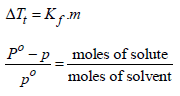Depression in freezing point, ΔTf = 0 - (-0.30) = 0.30
Now we know that ΔTf = Kfm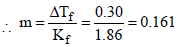According to Raoult’s law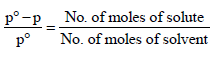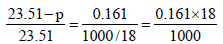(∵ No. of moles of H2 O = 1000/18)

On usual calculations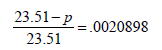p = 23.51– 23.51´0.0020898 = 23.51 – .068
p = 23.44 mm Hg

15. Nitrobenzene is formed as the major product along with a minor product in the reaction of benzene with a hot mixture of nitric acid and sulphuric acid. The minor product consists of carbon : 42.86%, hydrogen : 2.40% , nitrogen : 16.67%, and oxygen: 38.07% (i) Calculate the empirical formula of the minor product. (ii) When 5.5 g of the minor product is dissolved in 45 g of benzene, the boiling point of the solution is 1.84 °C higher than that of pure benzene. Calculate the molar mass of the minor product and determine its molecular and structural formula. (Molal boiling point elevation constant of benzene is 2.53 K kg mol–1.) (1999 - 10 Marks)
Ans :
168 g, C6H4 (NO2 )2 , m-dinitrobenzene

Solution :

TIPS/Formulae : ΔTb = kb ´ m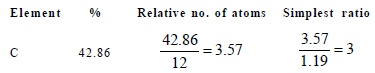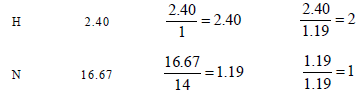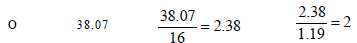∴Empirical formula of the minor product is C3H2NO2  Molar empirical formula mass of the minor prodouct = 3 × 12 + 2 × 1 + 1 × 14 + 2 × 16 = 84 g mol–2
Let M be the molar mass of the minor product. For 5.5 g of the minor product dissolved in 45 g benzene, the molality

(m) of the solution =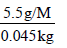Substituting this in the expression of elevation of boiling point,

ΔTb = Kbm ⇒ 1.84K = (2.53 Kmol-1)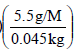or M = 168 g mol–1
No. of unit of empirical formula in molecular formula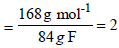Hence the molecular formula of the minor product is
2 (C3H2NO2), i.e., C6H4(NO2)2

The product is m - dinitrobenzene .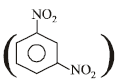16. To 500 cm3 of water, 3.0 × 10–3 kg of acetic acid is added. If 23% of acetic acid is dissociated, what will be the depression in freezing point? Kf and density of water are 1.86 K kg–1 mol–1 and 0.997 g cm–3, respectively. (2000 - 3 Marks)
Ans :
0.228 K

Solution :

TIPS/Formulae :

ΔTf = ixKf x m

Weight of water = 500 × 0.997 = 498.5 g
( weight = volume × denisty)
No. of moles of acetic acid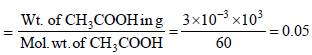Since 498.5 g of water has 0.05 moles of CH3COOH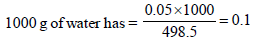Therefore molality of the solution = 0.1
Determination of van’t Hoff factor, i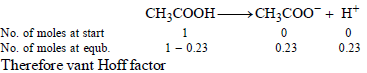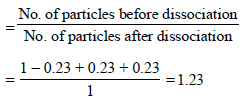Now we know that

ΔTf = ixKf xm = 1.23 x 1.86 x 0.1= 0.228K

17. 1.22g of benzoic acid is dissolved in 100 g of acetone and 100 g of benzene separately. Boiling point of the solution in acetone increases by 0.17º C, while that in the benzene increases by 0.13º C; Kb for acetone and benzene is 1.7 K kg mol–1 and 2.6 K kg mol–1. Find molecular weight of benzoic acid in two cases and justify your answer. (2004 - 2 Marks)
Ans :
122, 224

Solution :

TIPS/Formulae :
ΔTb = Kb × M
In first case,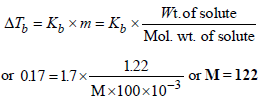Thus the benzoic acid exists as a monomer in acetone

(ii) In second case,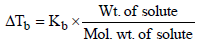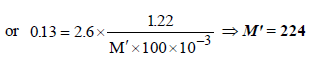NOTE : Double the expected molecular weight of benzoic acid (244) in acetone solution indicates that benzoic acid exists as a dimer in acetone.

18. 75.2 g of C6H5OH (phenol) is dissolved in a solvent of Kf = 14. If the depression in freezing point is 7 K then find the % of phenol that dimerises. (2006 - 6M)

Ans : 75%

Solution :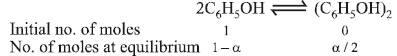Total number of moles at equilibrium =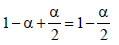ΔTf =iKf ×(molality)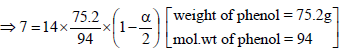∴ a = 0.75So the percentage of phenol that dimerises = 75%.

The document Subjective Type Questions: Solutions- 3 | JEE Advanced - Notes | Study Chemistry 35 Years JEE Main & Advanced Past year Papers - JEE is a part of the JEE Course Chemistry 35 Years JEE Main & Advanced Past year Papers.
All you need of JEE at this link: JEE

## Chemistry 35 Years JEE Main & Advanced Past year Papers

142 docs|66 tests

## Chemistry 35 Years JEE Main & Advanced Past year Papers

142 docs|66 tests

Track your progress, build streaks, highlight & save important lessons and more!

,

,

,

,

,

,

,

,

,

,

,

,

,

,

,

,

,

,

,

,

,

;